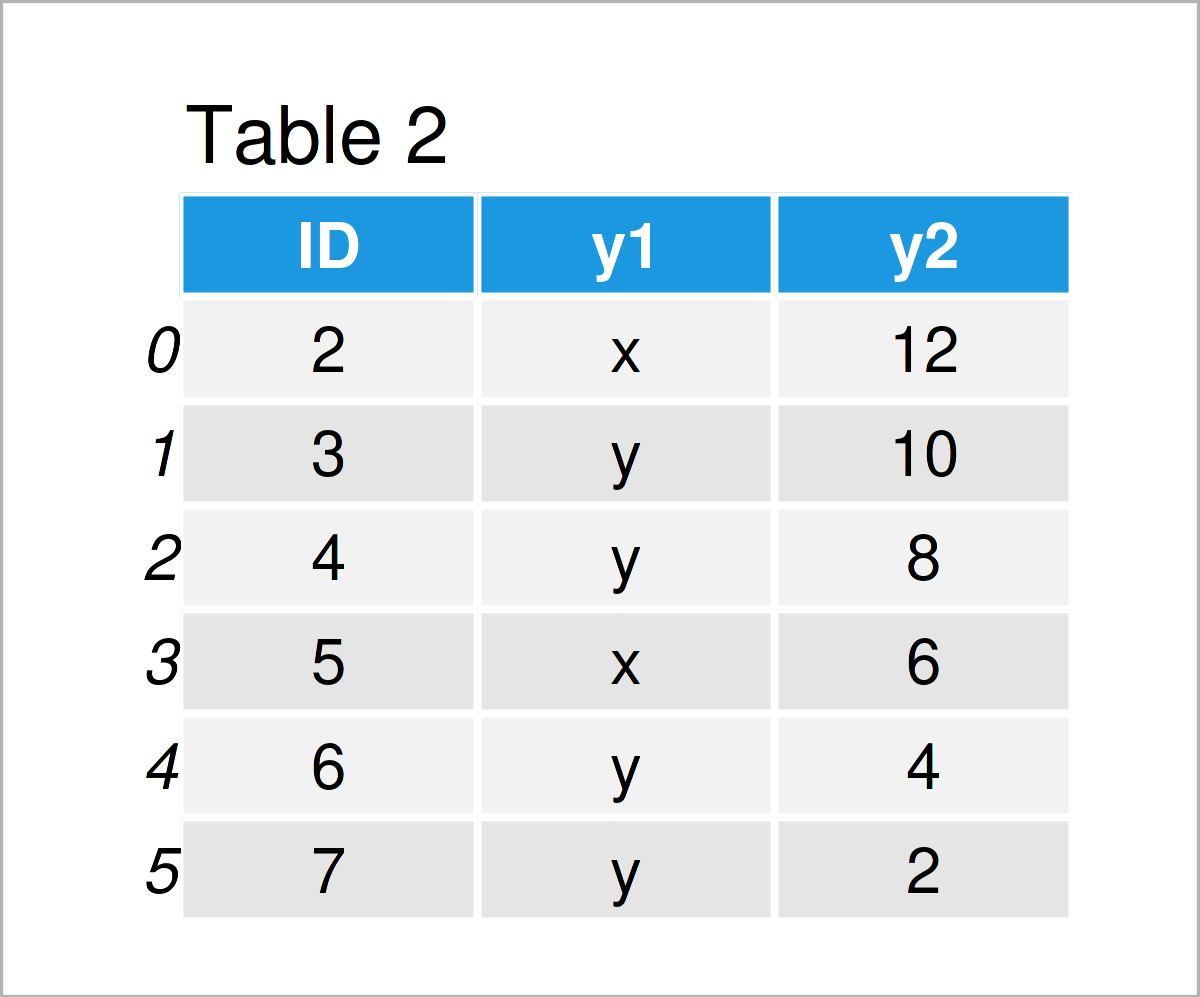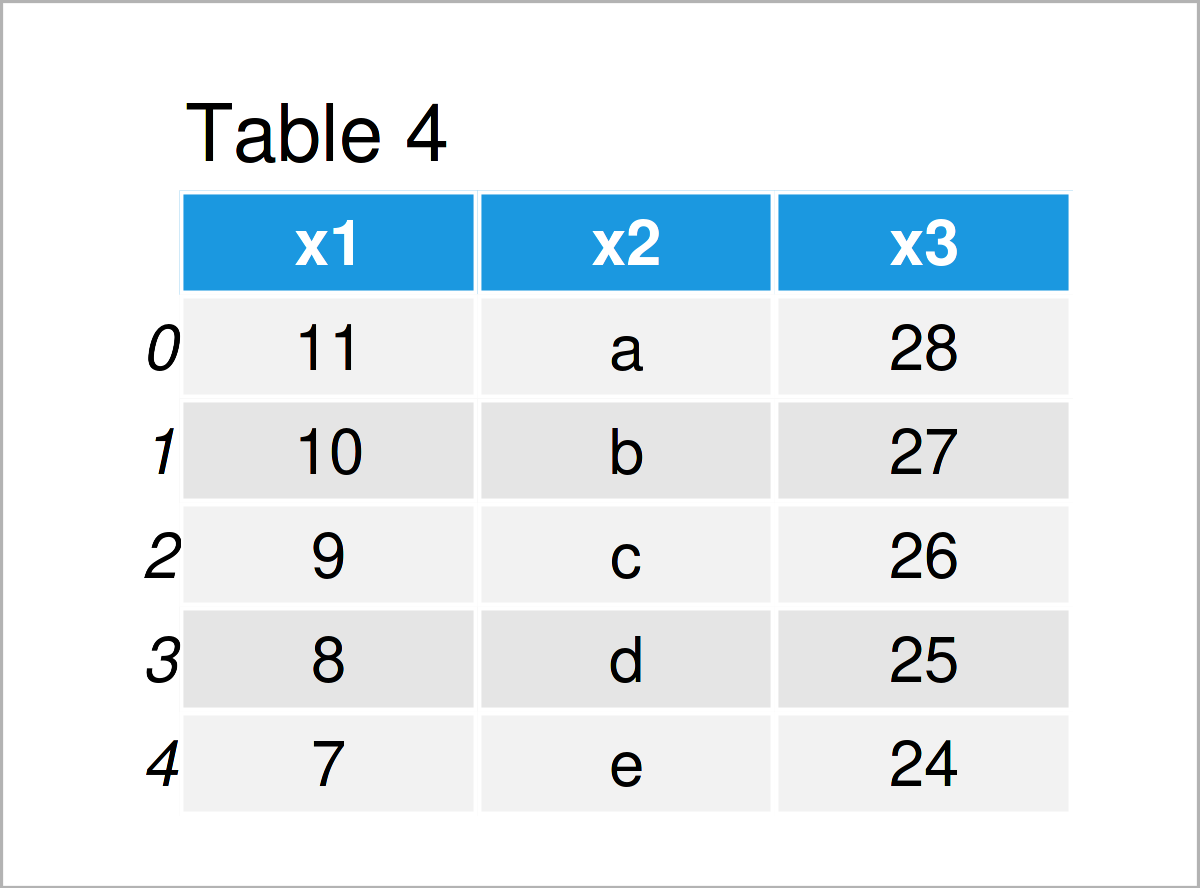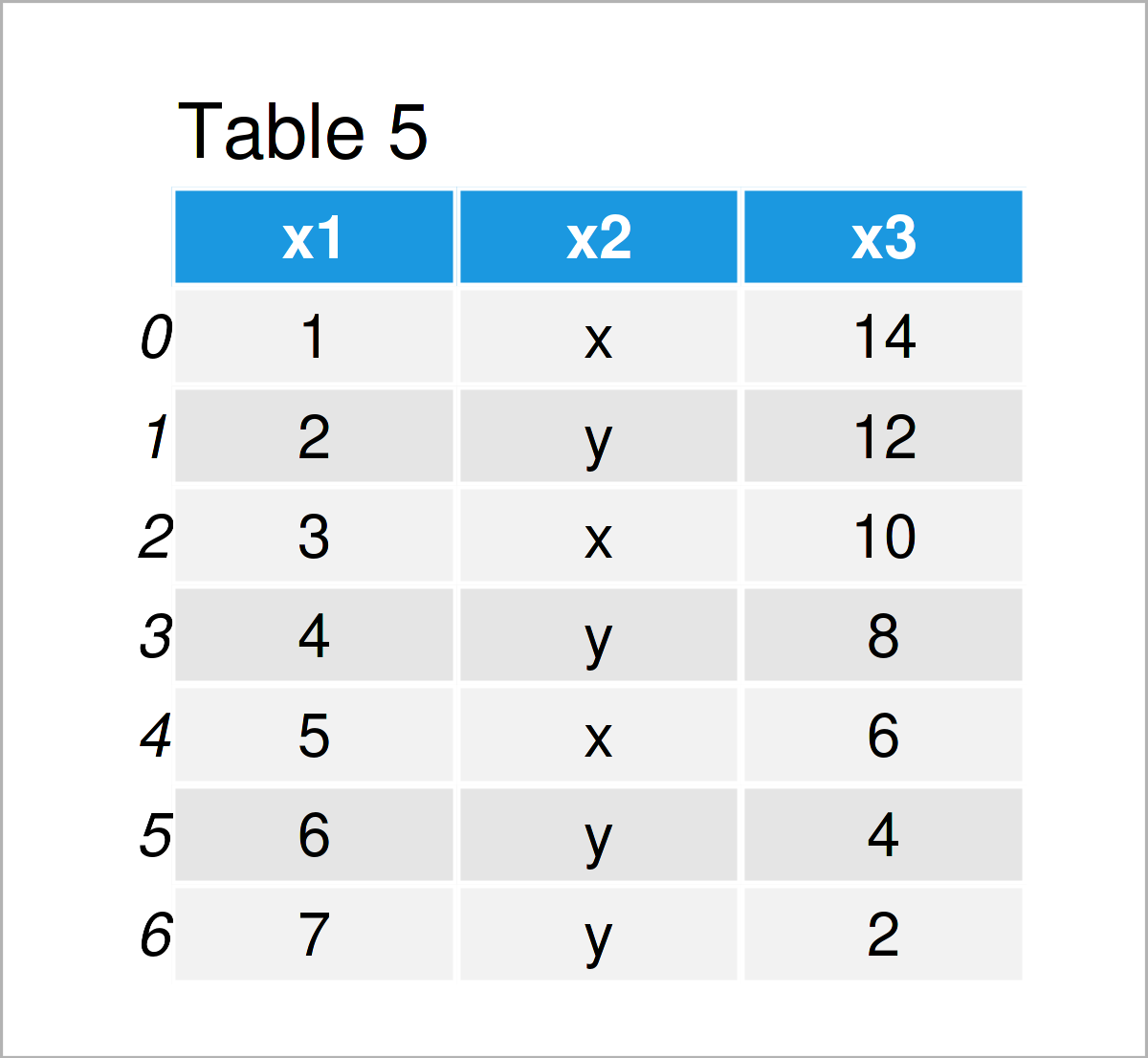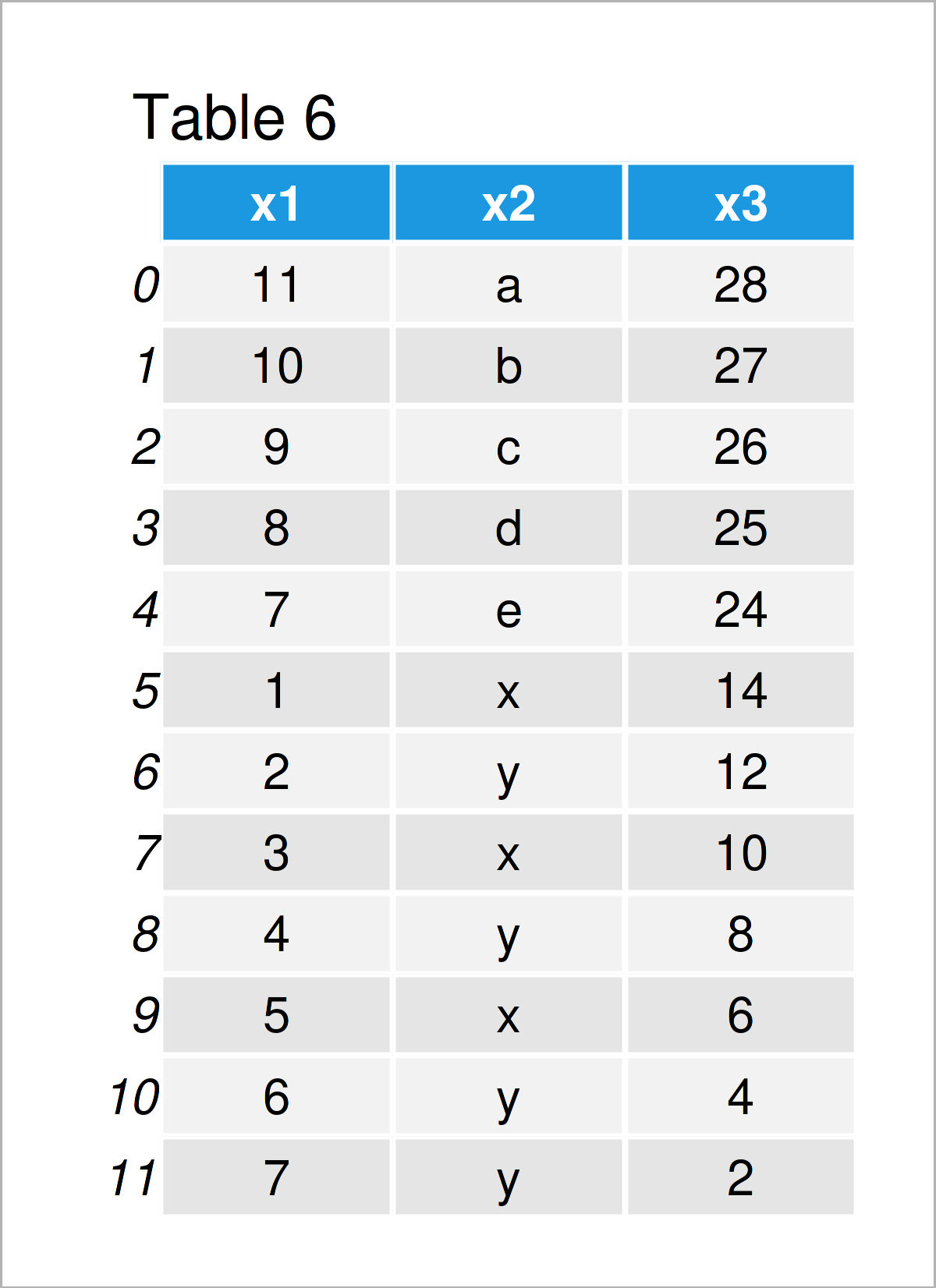# Combine pandas DataFrames Vertically & Horizontally in Python (2 Examples)

This article illustrates how to merge pandas DataFrames vertically and horizontally in the Python programming language.

Let’s get started:

## Example 1: Combine pandas DataFrames Horizontally

Example 1 explains how to merge two pandas DataFrames side-by-side.

To be able to apply the functions of the pandas library, we first need to import pandas:

`import pandas as pd                            # Load pandas library`

Next, we can construct two pandas DataFrames as shown below:

```data1a = pd.DataFrame({"ID":range(1, 5),       # Create first pandas DataFrame
"x1":range(10, 6, - 1),
"x2":["a", "b", "c", "d"],
"x3":range(26, 22, - 1)})
print(data1a)                                  # Print first pandas DataFrame``````data2a = pd.DataFrame({"ID":range(2, 8),       # Create second pandas DataFrame
"y1":["x", "y", "y", "x", "y", "y"],
"y2":range(12, 1, - 2)})
print(data2a)                                  # Print second pandas DataFrame```The output of the previous Python programming syntax is shown in Tables 1 and 2: We have created two pandas DataFrames with a shared ID column, but different variables and values.

If we want to concatenate these two data sets horizontally, we have to apply the merge function as shown below:

```data_horizontal = pd.merge(data1a,             # Combine horizontally
data2a,
on = "ID",
how = "outer")
print(data_horizontal)                         # Print merged DataFrame```The output of the previous Python code is shown in Table 3 – A horizontally appended pandas DataFrame containing the values of our two input DataFrames.

Note that we have used an outer join in this example. However, we could apply the same syntax to perform other types of joins such as inner, left, and right joins.

## Example 2: Combine pandas DataFrames Vertically

This example demonstrates how to append two pandas DataFrames vertically.

In preparation of the example, we first have two create two pandas DataFrames:

```data1b = pd.DataFrame({"x1":range(11, 6, - 1),
"x2":["a", "b", "c", "d", "e"],
"x3":range(28, 23, - 1)})
print(data1b)                                  # Print first pandas DataFrame``````data2b = pd.DataFrame({"x1":range(1, 8),       # Create second pandas DataFrame
"x2":["x", "y", "x", "y", "x", "y", "y"],
"x3":range(14, 1, - 2)})
print(data2b)                                  # Print second pandas DataFrame```Tables 4 and 5 show the output of the previous Python code: We have created two pandas DataFrames with the same column names.

We can now stack these two data sets on top of each other using the concat function:

```data_vertical = pd.concat([data1b, data2b],    # Combine vertically
ignore_index = True,
sort = False)
print(data_vertical)                           # Print combined DataFrame```By executing the previous syntax, we have created Table 6, i.e. a stacked version of our two input data sets.

## Video & Further Resources

Some time ago, I have published a video on my YouTube channel, which shows the topics of this article. Please find the video instruction below.

Please accept YouTube cookies to play this video. By accepting you will be accessing content from YouTube, a service provided by an external third party.If you accept this notice, your choice will be saved and the page will refresh.

Besides that, you could have a look at the related tutorials on my website:

To summarize: In this Python tutorial you have learned how to join and add two pandas DataFrames vertically and horizontally to create a data set union. Tell me about it in the comments, in case you have further questions.

Subscribe to the Statistics Globe Newsletter